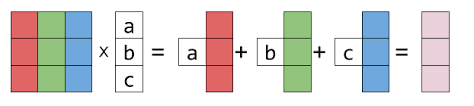Get inspired by the success stories of our students in IIT JAM MS, ISI  MStat, CMI MSc Data Science.  Learn More

# ISI MStat PSB 2010 Problem 1 | Tricky Linear Algebra QuestionThis is a very beautiful sample problem from ISI MStat PSB 2010 Problem 1 based on Matrix multiplication and Eigen values and Eigen vectors . Let's give it a try !!

## Problem- ISI MStat PSB 2010 Problem 1

Let $A$ be a $4 \times 4$ matrix with non-negative entries such that the sum of the entries in each row of $A$ equals 1 . Find the sum of all entries in matrix $A^{5}$ .

### Prerequisites

Matrix Multiplication

Eigen Values

Eigen Vectors

## Solution :

Doing this problem you have to use the hint given in the question . Here the hint is that the sum of the entries in each row of $A$ equals 1 . How can you use that ? Think about it!

Here comes the trick .

Let V be a vector such that $V={[1,1,1,1]}^{T}$ . Now if we multiply A by V then we will get V i.e $AV=V$ .

This is because it is given that the sum of the entries in each row of $A$ equals 1 .

So, from $AV=V$ we can say that 1 is an eigen value of A .

Hence $A^5V=A^4(AV)=A^4V=A^3(AV)= \cdots = V$ . From here we can say the sum of all the entries of each rows of $A^5$ is 1.

Therefore the sum of all the entries of $A^5$ is also 4 .

## Food For Thought

Let $A$ and B be $n \times n$ matrices with real entries satisfying $tr(A A^{T}+B B^{T})=tr(A B+A^{T} B^{T})$ .
Prove that $A=B^{T}$ .

Hint : Use properties of trace that's the trick here .

## Subscribe to Cheenta at Youtube

This is a very beautiful sample problem from ISI MStat PSB 2010 Problem 1 based on Matrix multiplication and Eigen values and Eigen vectors . Let's give it a try !!

## Problem- ISI MStat PSB 2010 Problem 1

Let $A$ be a $4 \times 4$ matrix with non-negative entries such that the sum of the entries in each row of $A$ equals 1 . Find the sum of all entries in matrix $A^{5}$ .

### Prerequisites

Matrix Multiplication

Eigen Values

Eigen Vectors

## Solution :

Doing this problem you have to use the hint given in the question . Here the hint is that the sum of the entries in each row of $A$ equals 1 . How can you use that ? Think about it!

Here comes the trick .

Let V be a vector such that $V={[1,1,1,1]}^{T}$ . Now if we multiply A by V then we will get V i.e $AV=V$ .

This is because it is given that the sum of the entries in each row of $A$ equals 1 .

So, from $AV=V$ we can say that 1 is an eigen value of A .

Hence $A^5V=A^4(AV)=A^4V=A^3(AV)= \cdots = V$ . From here we can say the sum of all the entries of each rows of $A^5$ is 1.

Therefore the sum of all the entries of $A^5$ is also 4 .

## Food For Thought

Let $A$ and B be $n \times n$ matrices with real entries satisfying $tr(A A^{T}+B B^{T})=tr(A B+A^{T} B^{T})$ .
Prove that $A=B^{T}$ .

Hint : Use properties of trace that's the trick here .

## Subscribe to Cheenta at Youtube

This site uses Akismet to reduce spam. Learn how your comment data is processed.

### Knowledge Partner